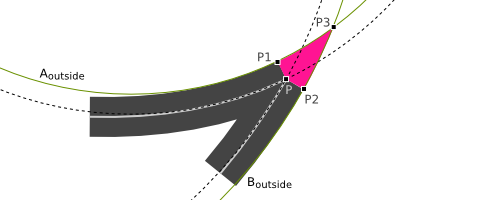# An Extrapolated Line Join Style

A Work in Progress — Last updated 1 Sept 2012

## The Problem

SVG as well as most other drawing languages have three line join styles: miter, round, and bevel. When a sharp join is desired, the miter style usually looks fine for straight line segments. However, when the two segments to be joined are curved, the miter join may look unnatural, especially when the angle between the segments is small as the curved edges of the path suddenly become straight in the miter. Recently, Inkscape has added a fourth option using a "Live Path Effect". This option results in the curved edges of the path being "extrapolated" until they intersect. It results in a much nicer join.A comparison of the different join styles including the proposed extrapolated style.

The SVG Working group discussed adding an extrapolated join style to the specification and there was wide spread support. A couple members asked that the exact mathematical formulas be spelled out. This web page aims to do just that.

The basic method illustrated here is to extrapolate the outer sides of the two intersecting lines using the curvatures at the point where the two lines are joined. The extrapolated sides are the just circular arcs with the calculated curvatures.

The signed curvature $$\kappa$$ of a line $$r$$ at a particular point $$t$$ is the rate of change of the tangent vector $$\vec T$$ with respect to the "speed" (arc length) of a particle moving at unit speed $$s$$ along the path. That is: $$\kappa = {{d\vec T}\over{ds}}.$$

A little bit of math shows that (see for example "curvature" entry in the Wikipedia): $$\kappa = {{\vec v \times \vec v'} \over |v|^3}$$ where the "velocity vector" is defined as $$\vec v(t) \equiv {d\over{dt}} \vec r(t).$$

For example, a cubic Bezier curve defined by four points $$P_1, P_2, P_3,$$ and $$P_4$$ is given by the equation $$\vec r(t) = (1-t)^3 P_0 + 3(1-t)^2t P_1 + 3(1-t)t^2 P_2 + t^3 P_3.$$ where $$t$$ ranges over the interval of 0 to 1.

Thus we have: $$\vec v(t) = -3(1-t)^2 P_0 + 3(-2(1-t)t + (1-t)^2) P_1 + 3(-t^2 + 2(1-t)t) P_2 + 3t^2 P_3$$ or $$\vec v(t) = 3(-(1-t)^2 P_0 + (1-4t+3t^2) P_1 + (2t-3t^2) P_2 + t^2 P_3$$ and $$\vec v'(t) = 6((1-t) P_0 - (2-3t) P_1 + (1-3t) P_2 + tP_3).$$

We need the curvatures only at the start and end of a path segment, i.e., at $$t=0$$ and $$t=1$$: $$\vec v(0) = 3(P_1-P_0)$$ $$\vec v(1) = 3(P_3-P_2)$$ $$\vec v'(0) = 6((P_0-P_1) + (P_2-P_1))$$ $$\vec v'(1) = 6((P_1-P_2) + (P_3-P_2))$$ $$\kappa(0) = {2\over3}{(P_1-P_0)\times((P_0-P_1)+(P_2-P_1))\over|P_1-P_0|^3}$$ $$\kappa(1) = {2\over3}{(P_3-P_2)\times((P_1-P_2)+(P_3-P_2))\over|P_3-P_2|^3}$$

Two circles are now constructed, one tangent to the end point of the incoming segment with same curvature as the segment at that point, and one tangent to the the start point of the outgoing segment with the same curvature as that segment at the start point. The center of each circle will be $$1/\kappa$$ from the corner point and in a direction orthogonal to the velocity vector. The sign of the curvature determines which side the center lies with respect to the segment.

Next, two new circles are constructed concentric with the previously constructed circles but with radii that are either half the stroke width larger or smaller depending on the direction of the curvature so that the new circles are tangent to the outside of the joined segments where the outside is defined to be on the reflex angle side formed by the tangents to the segments at the point of intersection. Note that if a curvature is zero, the circle is actually a line. This case can be handled by using a line that is shifted by half the stroke width in a perpendicular direction.

If the two circles intersect, the join is constructed using the circles to outline the region to be filled. The closest point where the circles intersect becomes the tip of the join.

If the two new circles don't intersect, an extrapolated join cannot be constructed, and the join style falls back to miter, if the miter-limit is not satisfied, the join falls back to bevel.Two Bezier path segments join at point P. The curvatures of both segments are determined at this point and are used to construct two circles (dashed) tangent to the segments at point P with the determined curvature. Two new circles (green) are constructed concentric to the initial circles with radii differing by half the stroke width. These new circles are used to define the fill area of the extrapolated line join.